# Class 10 NCERT Solutions- Chapter 3 Pair of Linear Equations in Two Variables – Exercise 3.7

• Last Updated : 03 Mar, 2021

### Question 1. The ages of two friends Ani and Biju differ by 3 years. Ani’s father Dharam is twice as old as Ani and Biju is twice as old as his sister Cathy. The ages of Cathy and Dharam differ by 30 years. Find the ages of Ani and Biju.

Solution:

The age difference between Ani and Biju = 3 yrs.

Attention reader! All those who say programming isn't for kids, just haven't met the right mentors yet. Join the  Demo Class for First Step to Coding Coursespecifically designed for students of class 8 to 12.

The students will get to learn more about the world of programming in these free classes which will definitely help them in making a wise career choice in the future.

Case 1: Either Biju is 3 years older than that of Ani,

y – x = 3

Case 2: or Ani is 3 years older than Biju.

x – y = 3

Given, Ani’s father’s age is 30 yrs more than that of Cathy’s age.

Let’s take,

Ani’s age = x

and Biju’s age = y

So, Dharam’s age will be = 2x

And the age of Biju sister (Cathy) will be =CASE 1 (y > x)

According to the given condition,

y – x = 3              -(1)

and, 2x−y/2 = 30

Multiply it by 2, we get

4x – y = 60              -(2)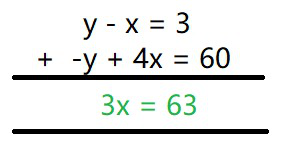3x = 63

x = 63/3

x = 21

Now putting x = 21 in eq(1), we get

y – 21 = 3

y = 24

Therefore, the age of Ani = 21 years

And the age of Biju is = 24 years.

CASE 2: (x > y)

According to the given condition,

x – y = 3               -(1)

and, 2x−y/2 = 30

Multiply it by 2, we get

4x – y = 60              -(2)

Now, by using Elimination method,

Subtract eq(1) from eq(2)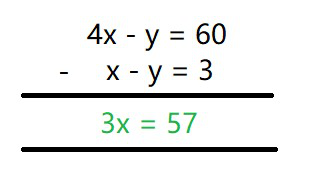3x = 57

x = 57/3

x = 19

Now putting x = 19 in eq(1), we get

19 – y = 3

y = 19 – 3

y = 16

Therefore, the age of Ani = 19 years

And the age of Biju is = 16 years.

### [Hint : x + 100 = 2(y – 100), y + 10 = 6(x – 10)].

Solution:

Let there be two persons as A and B.

Let’s take,

Money person A has = ₹ x

Money person B has = ₹ y

So, According to the given conditions, we have

x + 100 = 2(y – 100)

x – 2y  = – 300              -(1)

And

6(x – 10) = (y + 10)

6x -y = 70               -(2)

Now, by using Elimination method,

Multiply eq(2) by 2 and subtract eq(1) from it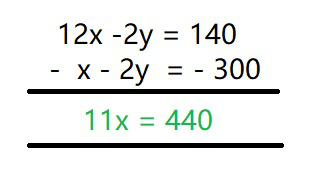11x = 440

x = 40

Now putting x = 40 in eq(1), we get

40 – 2y  = – 300

2y = 300 + 40

y = 340/2

y = 170

Hence,

Person A had Rs 40 and person B had Rs 170 with them.

### Question 3. A train covered a certain distance at a uniform speed. If the train would have been 10 km/h faster, it would have taken 2 hours less than the scheduled time. And, if the train were slower by 10 km/h; it would have taken 3 hours more than the scheduled time. Find the distance covered by the train.

Solution:

Let the speed of the train = x km/hr

The time taken by the train to travel a distance = t hours

The distance to travel = d km.

As we know,

Distance traveled by train = Speed of the train × Time taken to travel that distance

d = xt              -(1)

According to the given equation,

d = (x + 10) × (t – 2)

d = xt + 10t – 2x – 20

as d = xt, we get

10t – 2x = 20              -(2)

and 2nd condition,

d = (x – 10) × (t + 3)

d = xt – 10t + 3x – 30

as d = xt, we get

10t – 3x = -30               -(3)

Now, by using Elimination method,

Subtract eq(3) from eq(2), we get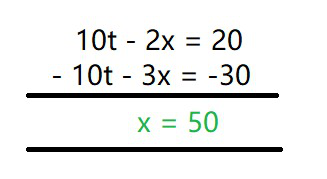x = 50 km/h

Now putting x = 50 in eq(1), we get

10t – 2(50) = 20

10t = 120

t = 12 hours

Now, distance will be = 50 × 12 = 600 km

Hence, the distance covered by the train is 600 km.

### Question 4. The students of a class are made to stand in rows. If 3 students are extra in a row, there would be 1 row less. If 3 students are less in a row, there would be 2 rows more. Find the number of students in the class.

Solution:

Let the number of rows = x

and, the number of students in a row = y.

Total number of students = Number of rows x Number of students in a row = xy              -(1)

Here, total no. of student will be same always

According to the given condition,

Total number of students = (x – 1)(y + 3)

xy = (x – 1)(y + 3)

xy = xy – y + 3x – 3

3x – y = 3               -(2)

and,

Total Number of students = (x + 2 ) ( y – 3 )

xy = xy + 2y – 3x – 6

3x – 2y = -6              -(3)

Now, by using Elimination method,

Subtract eq(3) from eq(2), we get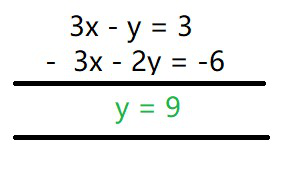y = 9

Now putting y = 9 in eq(1), we get

3x – 9 = 3

3x = 12

x = 4

Number of total students in a class = xy

= 4 x 9

= 36

Hence, No. of students = 36

### Question 5. In a ∆ABC, ∠C = 3 ∠B = 2 (∠A + ∠B). Find the three angles.

Solution:

Here, according to the given conditions

3 ∠B = 2 (∠A + ∠B)

3 ∠B = 2∠A + 2∠B

∠B = 2∠A              -(1)

and, ∠C = 3∠B

∠C = 3 (2∠A)

∠C = 6∠A               -(2)

As we know,

∠A + ∠B + ∠C = 180° (Angle sum property)

∠A + (2∠A) + (6∠A) = 180°          -(From eq(1) and eq(2))

9∠A = 180

∠A = 20°

∠B = 2∠A = 2(20°) = 40°

∠C = 6∠A = 6(20°) = 120°

Hence, the three angles are 20°, 40° and 120°.

### Question 6. Draw the graphs of the equations 5x – y = 5 and 3x – y = 3. Determine the coordinates of the vertices of the triangle formed by these lines and the y axis.

Solution:

5x – y = 5               -(1)

3x – y = 3               -(2)

y-axis, x = 0              -(3)

To get the co-ordinates of the vertices of the triangle formed, lets get there intersection point,

Intersection of eq(1) and (2)

Now, by using Elimination method,

Subtract eq(2) from (1), we get

2x = 2

x = 1

Now putting x = 1 in eq(1), we get

5(1) – y = 5

y = 0

So, Intersection of eq(1) and (2) vertices are (1, 0)

Now, Intersection of eq(1) and (3)

As x =0 substitutes it in eq(1), we get

5(0) – y = 5

y = -5

So, Intersection of (I) and (III) vertices are (0, -5)

Now, Intersection of eq(2) and (3)

As x =0 substitutes it in eq(2), we get

3(0) – y = 3

y = -3

So, Intersection of eq(2) and (3) vertices are (0,-3)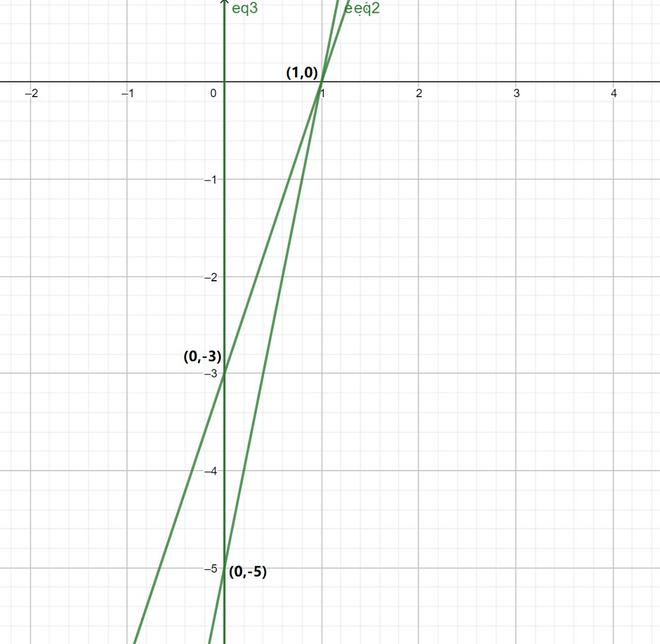Hence, the co-ordinates of the vertices of the triangle formed are (1, 0), (0, -5) and (0, -3)

### qx – py = p + q

Solution:

px + qy = p – q               -(1)

qx – py = p + q              -(2)

Multiplying p to equation (1) and q to equation (2), we get

p2x + pqy = p2 − pq              -(3)

q2x − pqy = pq + q2              -(4)

Add eq(3) and (4), we get

p2x + q2 x = p2  + q2

(p2 + q2) x = p2 + q2

x =x = 1

Now putting x = 1 in eq(1), we get

p(1) + qy = p – q

qy = p-q-p

qy = -q

y = -1

### bx + ay = 1 + c

Solution:

ax + by = c              -(1)

bx + ay = 1 + c              -(2)

Multiplying a to equation (1) and b to equation (2), we get

a2x + aby = ac              -(3)

b2x + aby = b + bc              -(4)

Subtract equation eq(4) from equation (3),

(a2 – b2) x = ac − bc– b

x =x =Now putting the value of x in eq(1), we get

ax + by = c+by = c+by = c

by =by =y =### ax + by = a2+ b2.

Solution:-(1)

Multiplying equation (1) with ab, we get

bx − ay = 0               -(new 1)

Multiplying a and b to equation (1) and (2) respectively, we get

b2x − aby = 0              -(3)

a2x + aby = a3 + ab3               -(4)

Add eq(3) and (4), we get

b2x + a2x = a3 + ab2

x (b2 + a2) = a (a2 + b2

x = a

Now putting x = a in eq(1), we get

b(a) − ay = 0

ab − ay = 0

ay = ab,

y = b

### (a + b)(x + y) = a2+ b2

Solution:

(a – b)x + (a + b) y = a2– 2ab – b2              -(1)

(a + b)(x + y) = a2+ b2              -(2)

Subtract equation (2) from equation (1), we get

(a − b) x − (a + b) x = (a2 − 2ab − b2) − (a2 + b2)

x(a − b − a − b) = − 2ab − 2b2

− 2bx = − 2b (b + a)

x = b + a

Substituting, x = b + a in equation (1), we get

(a + b)(a − b)  +y (a + b) = a2− 2ab – b2

a2 − b2 + y(a + b) = a2− 2ab – b2                            -(Using the identity (a + b)(a – b) = a2 – b2)

(a + b) y = − 2ab

y =### –378x + 152y = – 604

Solution:

x  =-(1)

− 378x + 152y = − 604

Diving it by 2, we get

− 189x + 76y = − 302              -(2)

Substitute x in equation (2), we get

−189+76y=−302

− (189)2y + 189 × 37 + (76)2 y = − 302 × 76

189 × 37 + 302 × 76 = (189)2 y − (76)2y

6993 + 22952 = (189 − 76) (189 + 76) y

29945 = (113) (265) y

y = 1

Using equation(1), we get

x =x = 152/76

x = 2

### Question 8. ABCD is a cyclic quadrilateral (see Figure). Find the angles of the cyclic quadrilateral.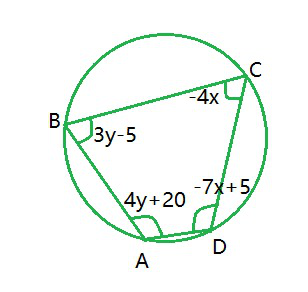Solution:

It is known that the sum of the opposite angles of a cyclic quadrilateral is 180°

Thus, we have

∠C +∠A = 180

4y + 20− 4x = 180

− 4x + 4y = 160

x − y = − 40              -(1)

And, ∠B + ∠D = 180

3y − 5 − 7x + 5 = 180

− 7x + 3y = 180              -(2)

Multiplying 3 to equation (1), we get

3x − 3y = − 120              -(3)

Adding equation (2) to equation (3), we get

− 7x + 3x = 180 – 120

− 4x = 60

x = −15

Substituting this value in equation (1), we get

x − y = − 40

-y−15 = − 40

y = 40-15

y = 25

∠A = 4y + 20 = 20 + 4(25) = 120°

∠B = 3y − 5 = − 5 + 3(25) = 70°

∠C = − 4x = − 4(− 15) = 60°

∠D = 5 – 7x

∠D= 5 − 7(−15) = 110°

Hence, ∠A, ∠B, ∠C and ∠D are equal to 120°, 70°, 60° and 110° respectively.

My Personal Notes arrow_drop_up